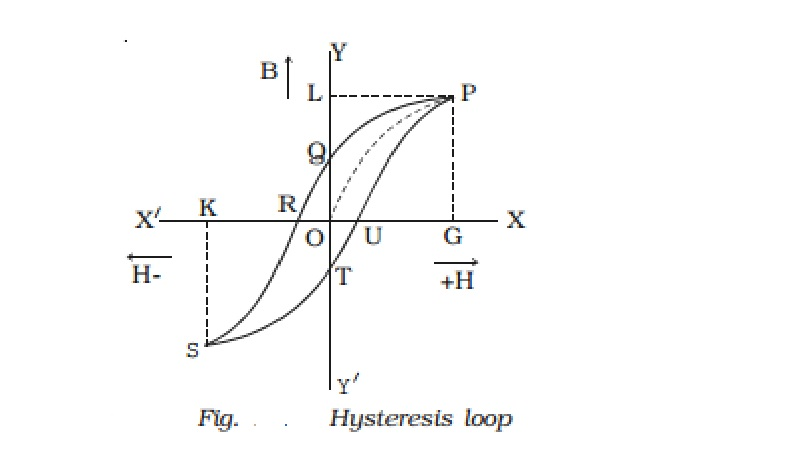Home | | Physics | Hysteresis and Hysteresis loss

# Hysteresis and Hysteresis lossConsider an iron bar being magnetised slowly by a magnetising field H whose strength can be changed. It is found that the magnetic induction B inside the material increases with the strength of the magnetising field and then attains a saturated level. This is depicted by the path OP in the Fig.

Hysteresis

Consider an iron bar being magnetised slowly by a magnetising field H whose strength can be changed. It is found that the magnetic induction B inside the material increases with the strength of the magnetising field and then attains a saturated level. This is depicted by the path OP in the Fig.

If the magnetising field is now decreased slowly, then magnetic induction also decreases  but it does not follow the path PO. Instead, when H = 0, B has non zero value equal to OQ. This implies that some magnetism is left in the specimen. The value of magnetic induction of a substance, when the magnetising field is reduced to zero, is called remanance or residual magnetic induction of the material. OQ represents the residual magnetism of the material. Now, if we apply the magnetising field in the reverse direction, the magnetic induction decreases along QR till it becomes zero at R. Thus to reduce the residual magnetism (remanent magnetism) to zero, we have to apply a magnetising field OR in the opposite direction.

The value of the magnetising field H which has to be applied to the magnetic material in the reverse direction so as to reduce its residual magnetism to zero is called its coercivity.When the strength of the magnetising field H is further increased in the reverse direction, the magnetic induction increases along RS till it acquires saturation at a point S (points P and S are symmetrical). If we now again change the direction of the field, the magnetic induction follows the path STUP. This closed curve PQRSTUP is called the ?hysteresis loop? and it represents a cycle of magnetisation. The word ?hysteresis? literally means lagging behind. We have seen that magnetic induction B lags behind the magnetising field H in a cycle of magnetisation. This phenomenon of lagging of magnetic induction behind the magnetising field is called hysteresis.

Hysteresis loss

In the process of magnetisation of a ferromagnetic substance through a cycle, there is expenditure of energy. The energy spent in magnetising a specimen is not recoverable and there occurs a loss of energy in the form of heat. This is so because, during a cycle of magnetisation, the molecular magnets in the specimen are oriented and reoriented a number of times. This molecular motion results in the production of heat. It has been found that loss of heat energy per unit volume of the specimen in each cycle of magnetisation is equal to the area of the hysteresis loop.

The shape and size of the hysteresis loop is characteristic of each material because of the differences in their retentivity, coercivity, permeability, susceptibility and energy losses etc. By studying hysteresis loops of various materials, one can select suitable materials for different purposes.

Study Material, Lecturing Notes, Assignment, Reference, Wiki description explanation, brief detail
11th 12th std standard Class Physics sciense Higher secondary school College Notes : Hysteresis and Hysteresis loss |